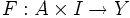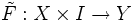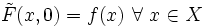# Cofibration

This article defines a property over pairs of a topological space and a subspace, or equivalently, properties over subspace embeddings (viz, subsets) in topological spaces

## Definition

A subspace$A$ of a topological space$X$ is said to be a cofibration, or to have the homotopy extension property if the following holds: given any map$f_0: X \to Y$ and a homotopy$F:A \times I \to Y$ such that$F(a,0) = f(a) \ \forall \ a \in A$, we have a homotopy$\tilde{F}:X \times I \to Y$ whose restriction to$A$ is$F$, and such that$\tilde{F}(x,0) = f(x) \ \forall \ x \in X$.

## Metaproperties

### Transitivity

This property of subspaces of topological spaces is transitive. In other words, if$A$ satisfies the property as a subspace of$B$ and$B$ satisfies the property as a subspace of$C$ then$A$ satisfies the property as a subspace of$C$